ABARIM
Publications
A Brief History of the End of Time—Where do Dimensions End?

# A Brief History of the End of Time

## 🔼The End of Any Dimension

Since Einstein discovered that time is nothing more than a dimension of space-time, we can imagine the End of Time by trying to find a general rule for all dimensions' ends. Things tend to get more complicated when things have more dimensions, so let' start with zero:

The simplest object we can (not) draw is a zero-dimensional object: a dot that has no size. Mathematically spoken, this dot should have a diameter that is equal to the smallest unit on the grid we are putting it on. In nature, this dot would have the Planck-length, and that is the smallest possible length (0.0000000000000000000000000000000016 centimeter). At scales smaller than the Planck length, quantum irregularity takes over and reality disappears in the mists of quantum foam (read our article on quantum foam).

We notice at once that when we put a dot on a piece of paper, the dot "ends" everywhere! We can clearly observe the boundaries of a zero-dimensional object by looking at it from the perspective of a two dimensional piece of paper.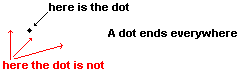Let's take a step up and look at a line that has no width (or the same width as the diameter of the dot). We notice that the line, which is a one-dimensional object, does not end in either direction. But it does end sideways. And, moreover, it ends in its every point!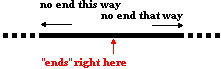The rule that we derive is that the end of a line exists in every point of the line, and in the direction not of the line. Or in other words: the end of a one-dimensional object is clearly visible when we look at the object from a perspective that has at least one dimension more.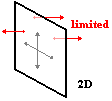Same with a two dimensional object: Even when there's no end in either of the two dimensions and either of the two directions (positive or negative), its edge is in every point, clearly visibly from outside the two dimensions in which it exists.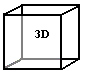Same as with a three dimensional object, like a cube: its edge is in every point within the cube.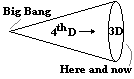Our space-time, which is a four dimensional object, ends not only in the past (namely in the Big Bang) and in the future (namely in the Heat Death, and that is the situation where all particles are so much dispersed that no relation exists and the entire universe regresses to a mere cloud of dots; read our article on the expansion of the universe), but also in every point within space-time. The End Of Time can be found in every point in space-time, provided we can look at it from outside of it.

At the speed of light all distances become zero and all time freezes. That means that the speed of light marks the end of space-time!

Suddenly, the cherub with the flaming sword at the entrance of Eden doesn't seem all that far-fetched anymore...

The End of Time cannot be observed from within time, but it can be observed from outside of it. Question is: what is outside space-time?

Go to the next chapter:
Things Outside Spacetime